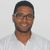QlikView App Dev

Discussion Board for collaboration related to QlikView App Development.

Announcements
QlikWorld 2022, LIVE in Denver CO., May 16-19, 2022. REGISTER NOW TO RECEIVE EARLY BIRD PRICING
cancel
Showing results for
Did you mean:Contributor II

Compare Loop Variable to Day(Date)

Hi I want to compare a Loop Variable to the Day of a Date Field.

When I hard code the Following Code is work Perfectly:

ExportFile_Future:
NoConcatenate
AEDO_transaction_id,
OPPS,
AM,
BFS_BRANCH_NAME,
EMPL_NAME,
AEDO_employer_code,
AEDO_TX_DATE_YEAR,
//num(tMonth) as tMonth
if(Month(AEDO_tran_date) = Month(AEDO_runout_date),
if(Day(AEDO_tran_date) <= (1) and Day(AEDO_runout_date) >= (1), 'x'),
if(Day(AEDO_tran_date) <= (1) or Day(AEDO_runout_date) >= (1), 'x')) as 1

But when I put it in a loop it returns a Null Value:

For iD = 1 to 4
Trace >>>>> Join Export File '\$(iD)' <<<<<;
//Temp:
//NoConcatenate
Left Join (ExportFile_Future)
AEDO_transaction_id,
OPPS,
AM,
BFS_BRANCH_NAME,
EMPL_NAME,
AEDO_employer_code,
'Future' as AEDO_TX_DATE_YEAR,
//tMonth,
IsNum(\$(iD)) as test,
if(Month(AEDO_tran_date) = Month(AEDO_runout_date),
if(Num(Day(AEDO_tran_date)) <= Num(\$(iD)) and Num(Day(AEDO_runout_date)) >= Num(\$(iD)), 'x'),
if(Num(Day(AEDO_tran_date)) <= Num(\$(iD)) or Num(Day(AEDO_runout_date)) >= Num(\$(iD)), 'x')) as \$(iD)
Resident AEDO
Where xml_transaction_type = 'AEDO_FUTURE';

Next iD

Labels (2)

• Variables

4 RepliesMVP

Hi,

Can you give us more details about the script or complete it?
for example what is the table "AEDO" in Resident, ... or send a sample data.

for example in this code "Left Join (ExportFile_Future)" while (ExportFile_Future) is still empty, it is normal that it returns nulls, each time we join with null.

send us a sample data so that it is easy to interpret the code

Taoufiq

Regards,
Taoufiq ZARRA

"Please LIKE posts and "Accept as Solution" if the provided solution is helpful "

(you can mark up to 3 "solutions") 😉Contributor II
Author

Code with Loop:

Trace Left Join Day Columns;
ExportFile_Future:
NoConcatenate
AEDO_transaction_id,
OPPS,
AM,
BFS_BRANCH_NAME,
EMPL_NAME,
AEDO_employer_code,
AEDO_TX_DATE_YEAR

Resident AEDO
Where xml_transaction_type = 'AEDO_FUTURE';

For iD = 1 to 4
Trace >>>>> Join Export File '\$(iD)' <<<<<;
//Temp:
//NoConcatenate
Left Join (ExportFile_Future)
AEDO_transaction_id,
OPPS,
AM,
BFS_BRANCH_NAME,
EMPL_NAME,
AEDO_employer_code,
'Future' as AEDO_TX_DATE_YEAR,
//tMonth,
IsNum(\$(iD)) as test,
if(Month(AEDO_tran_date) = Month(AEDO_runout_date),
if(Num(Day(AEDO_tran_date)) <= Num(\$(iD)) and Num(Day(AEDO_runout_date)) >= Num(\$(iD)), 'x'),
if(Num(Day(AEDO_tran_date)) <= Num(\$(iD)) or Num(Day(AEDO_runout_date)) >= Num(\$(iD)), 'x')) as \$(iD)
Resident AEDO
Where xml_transaction_type = 'AEDO_FUTURE';

Next iD

Drop Tables AEDO, Loans;
Exit Script;Contributor II
Author

Code without Loop:

(This one works but the I have to repeat code in bold x 31 Times)

Trace Left Join Day Columns;
ExportFile_Future:
NoConcatenate
AEDO_transaction_id,
OPPS,
AM,
BFS_BRANCH_NAME,
EMPL_NAME,
AEDO_employer_code,
AEDO_TX_DATE_YEAR,
//num(tMonth) as tMonth
if(Month(AEDO_tran_date) = Month(AEDO_runout_date),
if(Day(AEDO_tran_date) <= (1) and Day(AEDO_runout_date) >= (1), 'x'),
if(Day(AEDO_tran_date) <= (1) or Day(AEDO_runout_date) >= (1), 'x')) as 1

Resident AEDO
Where xml_transaction_type = 'AEDO_FUTURE';

Drop Tables AEDO, Loans;
Exit Script;MVP & Luminary

I suggest that you rethink your whole approach because it creates (with quite heavy join-loops) a crosstable which is usually very expensive to handle (in script and UI). Often there are better ways to create an appropriate datamodel and in your case it might be with the use of an IntervalMatch .

- MarcusCommunity Browser